Courses

# Chapter 2 (Part 1) BJT and FET - Notes, Basic Electronics, Electrical Engineering Electrical Engineering (EE) Notes | EduRev

## Electrical Engineering (EE) : Chapter 2 (Part 1) BJT and FET - Notes, Basic Electronics, Electrical Engineering Electrical Engineering (EE) Notes | EduRev

The document Chapter 2 (Part 1) BJT and FET - Notes, Basic Electronics, Electrical Engineering Electrical Engineering (EE) Notes | EduRev is a part of the Electrical Engineering (EE) Course Electrical Engineering SSC JE (Technical).
All you need of Electrical Engineering (EE) at this link: Electrical Engineering (EE)

BJT and FET

BIPOLAR JUNCTION TRANSISTOR
BJT: Bipolar Transistor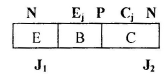collector region width is maximum
a) To collect maximum number of charge carrier.
b) To reduce heat dissipation. (per unit area surface).
Depletion layer in collector junction base side is maximum Emitter junction. Emitter side is minimum.

Biasing and application

 Input Jn J1  (EBjn ) Output Jn J2  (CB jn) Region in Which BJT works Application FB RB Active Amplifier FB FB Saturation BJTisOn orOFF RB RB Cut off OFF RB FB Inverted (Active) amplifier with decrease gain , b
• For Normal Biasing (i.e. Emitter junction FB and Collector junction RB) the emitter junction has low resistance.

Early Effect

For active region operation of transistor collector base region is reversed biased. Due to reverse bias the depletion width in base region increases which causes effective width of base to decrease. This effect is known as early effect or Base width modulation.
If the reverse bias Vcb is increased beyond a point, breakdown may occur as width of base becomes zero and phenomena is called punch through.

The physical behavior of Bipolar Transistor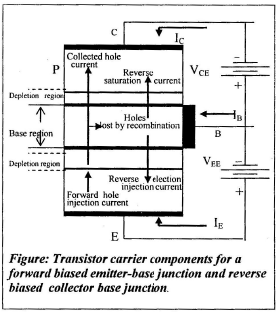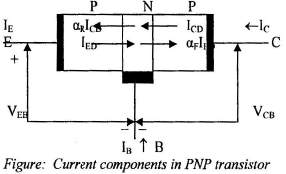On the basis of the consideration in the preceding paragraph we construct the Ebers-Moll mode in below figure. The two back to back diode (whose cathodes are connected) represent the junctions of the bipolar transistor, whereas the two controlled sources indicate the coupling between junctions.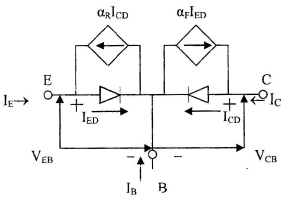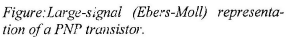The current IED and ICD are related to VEB and VCB by the diode volt ampere relation given in the equation. Thus the IE and IC can be expressed in terms of the two diodes current as
IE = IED – aR ICD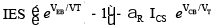IC =– aF IED + ICD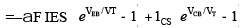The relationship expressed in above two equations are known s the Ebers-Moll equations.
The quantities IES and ICS in above equation are reverse saturation current of emitter base and reverse saturation current of emitter base and collector base junction, respectively. aF and aR are each less than unity as not all the current from one diode is couple to the other junction. The subscript refer to forward (F) transmission from emitter to collector and reverse (R) transmission from collector to emitter. aF IES = aR ICS and IB =– (IE + IC)

IE =– IES (eVEB/VT–1) + aR ICS (eVCB/VT– 1)
IC = aFIES (eVEB/VT–1)–ICS (eVCB/VT–1)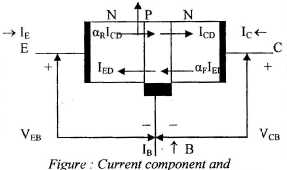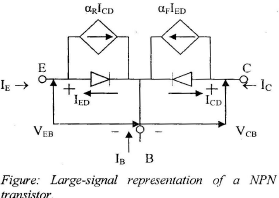FIELD EFFECT TRANSISTOR

Two Types : n channel and P channel.
It consists of a semiconductor block which is n type for n channel and P type for P channel. On both sides of n block acceptor impurities p are heavily doped by allowing or by diffusion. The region is called the gate. Ohmic contact are attached to the either ends of the n- block. One end is called source and other is called Drain. The np region between source and gate is reverse biased. In the vicinity of np junction there is diffusion of majority carriers, electrons and holes leaving uncover immobile positive  and negatives ions when RB is applied, the apace charge width increases uncovering more ions, until the space charge region from the two end, meets the n type region between the  two (p+) gates is called n channel and when a positive voltage is applied between drain and source, there is a current flow in the channel of majority carriers, (electrons). When a reverse bias is applied between the gate and source, constrication of the channel takes place by widening of the space charge depletion region controlling the flow of electrons to the drain.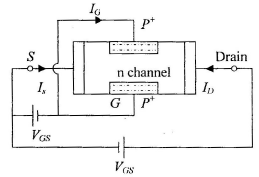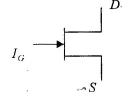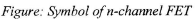Note: For a P channel FET the bar is a P type semiconductor and gate is dopped by n+ donor impurity.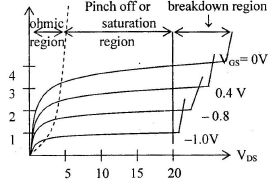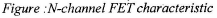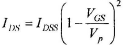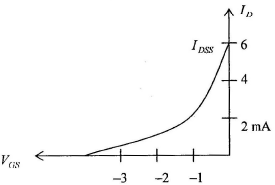1. Drain resistance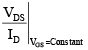Output resistance of FET and evaluated in constant drain current region of VDS –ID curve. It is of the order of 10 to 20 kWs.

2. Transconductance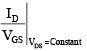it will be of the order of 2 k to 6 k micromhos.

3. Amplification Factor m = g mrd

4.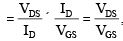It range of 40 to 100.

5. Input resistance rGS =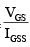It is of the order of hundreds of megaohmes.
FET is high impedance device.

Various Relationship FET

Pinch off voltage VP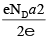2a ® Width of channel without any gates bias. 2b ® Width of channel after bias.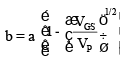when VGS = 0, b = a

If w ® channel dimension perpendicular to b direction

Drain current ID = Aq ND mn E
= 2bwq NDmn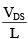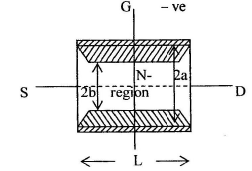Substituting ‘b’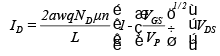The ON resistance rd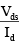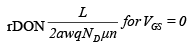Transfer Characteristics
In amplifier application, the FET is almost used in the region beyond pinch off (also called the  constant saturation region).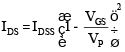Trans-conductance: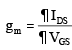Relation between gm, I DDS and IDS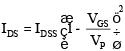(1)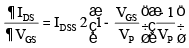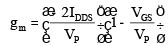(2)

gmo = it is value of gm for VGS = 0 and given by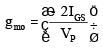(3)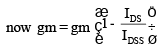(4)
From equation (i)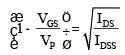From equation (2) and (5) From the transfer characteristic we have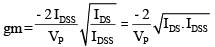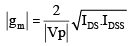BJT FET High voltage gain Low voltage gain Low current gain High current gain Lowinput and Output impedance High input andOutput impedance Mediumnoisegenertion g Low noise generation (Gain Band width) m (Gain Band width ) medium Current source Voltage controlled

Offer running on EduRev: Apply code STAYHOME200 to get INR 200 off on our premium plan EduRev Infinity!

65 docs|37 tests

,

,

,

,

,

,

,

,

,

,

,

,

,

,

,

,

,

,

,

,

,

,

,

,

,

,

,

;